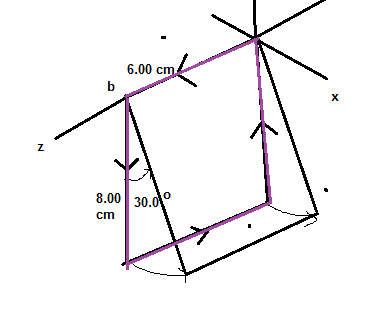# The rectangular loop of wire shown in the figure has a mass of 0.10 g per centimeter of length...

## Question:

The rectangular loop of wire shown in the figure has a mass of {eq}0.10\ g {/eq} per centimeter of length and is pivoted about side ab on a frictionless axis. The current in the wire is {eq}9.0\ A {/eq} in the direction shown.(a) Find the magnitude of the magnetic field parallel to the y-axis that will cause the loop to swing up until its plane makes an angle of {eq}30.0 ^\circ {/eq} with the yz-plane.Express your answer using two significant figures.

(b) Find the direction of the magnetic field parallel to the y-axis that will cause the loop to swing up until its plane makes an angle of {eq}30.0 ^\circ {/eq} with the yz-plane.

## Torque:

The torque on a particle about a position is equal to the vector product between the position vector and the force vector. For a loop of wire in a magnetic field, the torque experience by the loop is proportional to the current and the magnitude of the magnetic field.

Become a Study.com member to unlock this answer! Create your account

The mass of the loop of wire is:

{eq}M = (1.0\times10^{-4})(12+16) \\ M = 0.0028 \ kg {/eq}

The torque due to gravity is :

{eq}T =...Torque: Definition, Equation & Formula

from

Chapter 4 / Lesson 11
565K

You've probably heard of torque before, perhaps while discussing cars. Now learn what it really is and what it has to do with rotational equilibrium. Then, work through an example problem that combines the two.